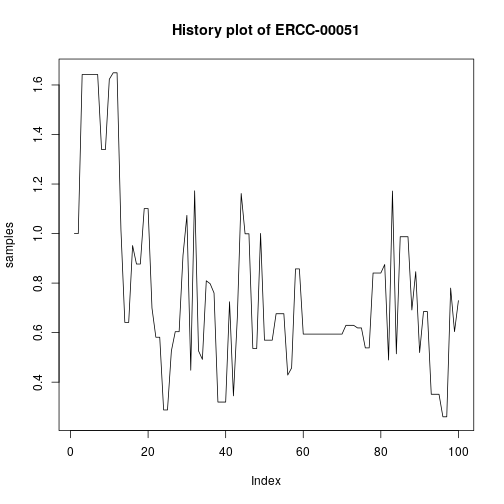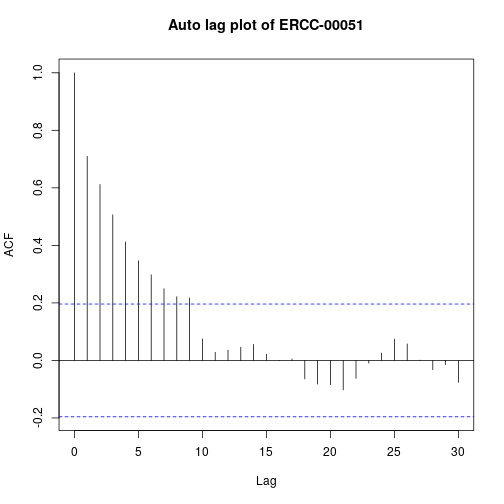##Introduction In this vignette, we give a brief introduction to the denoiSeq package for differential expression analysis of RNA-Seq and similar data. The statistical details of the methods used can be found in the associated publication.

The denoiSeq package is based on a model for PCR sequencing developed by @ndifon2012chromatin. The model generates, in a bottom-up manner, a probability distribution for the final copy number of a gene, which is in the form of a superposition of the negative binomial and the binomial distributions. Throughout this text, the word gene is used synonymously with any genomic attribute such as exons, transcripts, peptide sequences, etc. The derived distribution has three main parameters, i.e $$N, p$$ and $$f$$, which represent the initial gene amount before amplification, the amplification efficiency and the dilution rate, respectively. We then use Bayesian inference to estimate the model parameters. Uniform priors are chosen for parameters $$p$$ and $$f$$ and a non informative prior is chosen for $$N$$.

The package only performs a two group comparison and assumes that the user has the data in the form of an $$m$$ by $$n$$ matrix of integers that contains counts from both groups. We prefer that the matrix have atleast the rownames but this is not necessary. Each $$i,j$$ element of this matrix corresponds to counts for gene $$i$$ in sample $$j$$, whereby $$j$$ has been derived from one of the two experimental groups (conditions), i.e A and B. For an $$m$$ by $$n$$ matrix, inference aims at estimating the three sets of parameters, i.e $$p$$, $$f$$ and $$N_i$$'s ($$2m$$ in total because we are considering 2 conditions with the same $$m$$ genes in each). In the analysis, the counts from different experimental samples are first normalised using estimated size factors [@wolf] in order to make them comparable. We then perform differential analysis to determine if there is a signal of a significant difference between the counts for each gene (the same row) in the two conditions. For this kind of analysis, the primary parameters of interest are $$N_{iA}$$ and $$N_{iB}$$, for each gene $$i$$.

##Preparation We begin the analysis by creating a readsData object to handle the count data. This object has six slots namely; counts, geneNames, replicates, initValues, stepSize and output. The first four slots are specified before the analysis and the latter (output) is used to store the results of the Bayesian inference and is set by the results function. Briefly, the counts slot contains the matrix of counts; geneNames contains the names of the genes; replicates contains a list of two elements ($$A$$ and $$B$$), each of which is a vector of indices for the columns that belong to each respective condition; initValues contains the initial values; and stepSize contains the step sizes for sampling all parameters to be estimated.

Before we can create the readsData object, we first load the package.

library(denoiSeq)

This package is distributed with two datasets, i.e ERCC and simdat. The ERCC dataset contains RNA-seq data of biological replicates characterized during the ENCODE project [@djebali2012landscape] and simdat contains simulated data. In this first example, we will use the simdat dataset. This dataset contains 750 genes with 5 experimental samples for each condition, summarised as a 750 by 10 integer matrix. The first 428 genes are not differentially expressed between the two conditions whereas the last 322 genes are. The genes counts were generated in accordance to the probability distribution derived by @ndifon2012chromatin. We can examine the counts of the first and last 6 genes of this dataset using the head and tail commands in R respectively.

tail(simdat)

Because Bayesian inference is computationally intensive, users are advised to perform pre-filtering before creating the readsData object, in order to save time. An example would be

simdat <- simdat[ rowSums(simdat) > 0, ],

to eliminate the non-expressed genes. <!–since as has been reported [@bullard2010evaluation], it is difficult to detect accurately a signal of difference in such genes.–> This also helps to increase the computational efficiency of the analysis. <!–Threshold values of 30 for large datasets (> 400 genes) and 15 for smaller datasets (<=100 genes) were chosen based on analysis of existing annotated real datasets.–>

From the output of head() above, we notice that the simdat count matrix has no gene names and so we have to explicitly specify the geneNames slot. We begin by creating gene names below:

genenames <- paste("gene_", 1:750, sep = "_").

We then create a readsData object:

RD <- new("readsData", counts = simdat, geneNames = genenames)

With the exception of output, all the other remaining slots are set to default values. See the class documentation for more details using ?readsData. In the event that the object has already been formed, the default values can be altered using the class methods setReplicates, setInitValues and setStepSizes respectively.

##Bayesian inference Once the readsData is in place, we implement Bayesian inference by calling the denoiseq function, specifying the number of steps to be used for posterior sampling, and a smaller number steps to be used for step size tuning.

steps <- 30 #30 steps are just for illustration here. Atleast 5000 steps are adequate.

tuningSteps <- 10
BI <- denoiseq(RD, steps, tuningSteps)

The denoiSeq function then returns the same readsData object, but with a filled output slot.

In this example, the above chunk of code ran for about 1.5 minutes on a core i5, 8GB memory PC. We note that 30 steps is a very small number of iterations to obtain adequately accurate results. This piece of code is just meant for instruction purposes. At least 5000 steps should be adequate in this example, with about a third of those used for step size tuning (takes over an hour on the same computer). A smaller dataset is used in the case study section below.

##Differential analysis The results of the inference are used to determine differential expression. This is achieved by calling the results function, which returns a data frame with three columns, i.e the log2 fold change (log2FC), the standard error of the log2 fold change (lgfcSE) and the test static (ROPE_propn), and with as many rows as the number of genes.

The results function has the same arguments as denoiseq, with an additional rope_limits argument which is defaulted to 0.5. This parameter represents the upper bound of the log (to base 2) of the fold change that we consider non-significant.

rez <- results(BI, steps, tuningSteps)

##Other functions For diagnostics about the behavior of the posterior samples generated, the package has the getSamplesOf function that takes as argument, the name of a model parameter (i.e $$p,f$$,or the gene name for any of the $$N_i$$'s) and returns a vector of posterior samples for that parameter. The other arguments are a readsData object with a populated output slot, the number of steps used in sampling and the experimental condition being considered. Instead of a gene name, the function can also take the row index of the counts matrix. In the following example, we extract the posterior samples for the parameter $$N_{50}$$ (i.e the initial count of the $$50 ^{th}$$ gene) from condition $$B$$.

N_50 <- getSamplesOf(BI, "gene_50", steps, condition = "B")

With these posterior samples, diagnostics such as evaluating the acceptance rate, creating a history plot, and determining the autocorrelation between posterior parameter samples can be performed, as illustrated below:

acceptance_rate <- length(unique(gene_50)) / steps
plot(gene_50, type="l", main = "History plot of N_50")
AL <- acf(gene_50, lag.max = 30, type = c("correlation"), plot = T)

If one wishes, one can also have a look at the tuned step sizes using tunedStepSize. This function takes the readsData object returned by denoiseq as its only argument.

SS <- tunedStepSize(BI)

The returned value can then be assigned to the stepSize slot for use in another run of Gibbs sampling implemented with denoiseq.

##Case study: Analysis of the ERCC dataset In this case study, we will use the ERCC dataset which contains a mixture of 92 spike-in synthetic oligonucleotides that are mixed into experimental samples A and B at four mixing ratios: ½, 2/3, 1 and 4. The genes with a mixing ratio of 1:1 (rows 24 to 46), represent the only genes with non-significant expression differences between conditions A and B. There are five experimental samples for each condition.

###Data preview

dim(ERCC)
##  92 10
A_1 A_2 A_3 A_4 A_5 B_1 B_2 B_3 B_4 B_5
ERCC-00130 220103 182768 140678 268448 181856 66630 71108 77412 65282 64374
ERCC-00004 45385 32634 28388 52615 37544 12005 12531 14706 11119 11394
ERCC-00136 6650 4778 4185 7700 5882 1749 1786 2238 1694 2377
ERCC-00108 16725 14520 10626 20273 11323 4903 5544 5792 4963 3258

###Pre-filtering We pre-filtered the dataset by selecting rows with a rowsums above 0.

ERCC <- ERCC[rowSums(ERCC) > 0, ]
dim(ERCC)
##  92 10

###Creating the readsData object Since the count matrix has row names, these were automatically assigned to the geneNames slot.

reps <- list(A=c(1,2,3,4,5), B=c(6,7,8,9,10))#specifying the columns for each condition
m <- dim(ERCC)
initvalues <- list(N_A = rep(1, m), N_B = rep(1, m), p = 0.0001, f = 0.01)
stepsizes <- list(stepsizeN_A = rep(1, m), stepsizeN_B = rep(1, m)
, stepsize_p = 5e+07, stepsize_f = 1e3)
RD2 <- new("readsData", counts = ERCC, replicates = reps, initValues
= initvalues, stepSizes = stepsizes)

###Bayesian inference The tuningSteps argument has been set to the default value = floor(steps / 3).

steps <- 100#100 steps are just for illustration here. Atleast 5000 steps are adequate.

BI2 <- denoiseq(RD2, steps)

NOTE: A more adequate value for the number of steps is 5000 and this took about 16 minutes on the same PC.

###Differential expression analysis In this case, we will explicitly set the value for the 'rope_limits' argument.

rope = 0.5
rez2 <- results(BI2, steps, rope_limit = rope)
log2FC(B / A) lfcSE ROPE_propn
ERCC-00130 0.2263791 0.0026065 0.0000000
ERCC-00004 0.2139779 0.0033292 0.0000000
ERCC-00136 0.2386529 0.0078052 0.0000000
ERCC-00108 0.2478648 0.0049706 0.0000000
ERCC-00116 0.3540129 0.0149003 0.0149254
ERCC-00092 0.2835223 0.0113460 0.0000000

A threshold value of the statistic is then chosen to determine significance. One can be determined by arranging the ROPE_propn column in ascending order and selecting the most differentially expressed genes. Values ranging from 0.00075 to 0.4 were obtained for the Youden's index [@youden1950index] for a number of annotated datasets. The Youden's index is a statistic that captures the performance of a binary classifier. It is defined for all points of an ROC curve and its maximum value may be used as a criterion for selecting the optimum cut-off point when a diagnostic test gives a numeric rather than a binary result.

#Re-ordering according to most differentially expressed
rez3 <- rez2[with(rez2, order(ROPE_propn)), ]

In this case, we chose a value of 0.38.

#Determine significant genes
sgf <- rez2[rez2\$ROPE_propn < 0.38, ]
dim(sgf)

Diagnostics

Posterior samples of parameter $$N$$ for gene $$ERCC-00051$$ in condition A are obtained and examined below, for the perfomance of Gibbs sampling.

samples <- getSamplesOf(BI2, "ERCC-00051", steps)
acceptance_rate <- length(unique(samples)) / steps
acceptance_rate
##  0.56

The history plot and autocorrelation function can then be plotted as shown below.

plot(samples, type="l", main = "History plot of ERCC-00051")
AL <- acf(samples, main = "Auto lag plot of ERCC-00051", lag.max = 30,
type = c("correlation"), plot = T)##session info

sessionInfo()
## R version 3.3.3 (2017-03-06)
## Platform: x86_64-pc-linux-gnu (64-bit)
## Running under: AIMS Desktop 2017.1
##
## locale:
##   LC_CTYPE=en_ZA.UTF-8       LC_NUMERIC=C
##   LC_TIME=en_ZA.UTF-8        LC_COLLATE=C
##   LC_MONETARY=en_ZA.UTF-8    LC_MESSAGES=en_ZA.UTF-8
##   LC_PAPER=en_ZA.UTF-8       LC_NAME=C
##  LC_MEASUREMENT=en_ZA.UTF-8 LC_IDENTIFICATION=C
##
## attached base packages:
##  stats     graphics  grDevices utils     datasets  methods   base
##
## other attached packages:
##  denoiSeq_0.1.1 knitr_1.15.1
##
## loaded via a namespace (and not attached):
##   backports_1.1.1 magrittr_1.5    rprojroot_1.2   htmltools_0.3.5
##   tools_3.3.3     Rcpp_0.12.10    stringi_1.1.2   rmarkdown_1.6
##   highr_0.6       stringr_1.2.0   digest_0.6.12   evaluate_0.10

##References